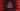# C++ program to solve a quadratic equation in different ways## C++ program to solve a quadratic equation:

In this post, we will learn how to find the roots of a quadratic equation in C++. The program will take the coefficients of the equation as inputs from the user and calculate the roots of the equation.

We can have real or complex roots based on the value of the discriminant. Let’s learn how to solve a quadratic equation and how to solve it in C++.

### How to solve a quadratic equation:

A quadratic equation with coefficient a, b and c looks as like below:

``ax^2+bx+c = 0``

It’s roots are:

``````-b + √ (b^2 - 4ac)/2ac

-b - √ (b^2 - 4ac)/2ac``````

Here, b^2 - 4ac is the discriminant.

• If the value of discriminant is positive, the roots will be real and different.
• If the value of discriminant is 0, the roots will be equal.
• If the value of discriminant is negative, the roots will be complex and different.

### C++ program to find the roots of a quadratic equation:

The following program will take the values of discriminants as inputs from the user and it will print the roots of the equation:

``````#include <iostream>
#include <cmath>
using namespace std;

int main()
{
float a, b, c;

cout << "Enter the values of a: " << endl;
cin >> a;

cout << "Enter the values of b: " << endl;
cin >> b;

cout << "Enter the values of c: " << endl;
cin >> c;

float d = b * b - 4 * a * c;

if (d == 0)
{
float root = -b / (2 * a);
cout << "First root: " << root << endl;
cout << "Second root: " << root << endl;
}
else if (d > 0)
{
float root1 = (-b + sqrt(d)) / (2 * a);
float root2 = (-b - sqrt(d)) / (2 * a);

cout << "First root: " << root1 << endl;
cout << "Second root: " << root2 << endl;
}
else
{
float real = -b / (2 * a);
float imaginary = sqrt(-d) / (2 * a);

cout << "First root: " << real << "+" << imaginary << "i" << endl;
cout << "Second root: " << real << "-" << imaginary << "i" << endl;
}
}``````
• The program asks the user to enter the values of a, b and c. It saves the values in the float variables a, b and c.
• The discriminant is calculated and stored in the variable d.
• Based on the discriminant value, the roots are calculated using the algorithm we discussed before.
• If d is equal to 0, roots are equal. If d is greater than 0, roots are different and positive and if d is negative, roots are different and imaginary.

If you run this program, it will give output as like below:

``````Enter the values of a:
12
Enter the values of b:
2
Enter the values of c:
3
First root: -0.0833333+0.493007i
Second root: -0.0833333-0.493007i

Enter the values of a:
4
Enter the values of b:
6
Enter the values of c:
0
First root: 0
Second root: -1.5``````

### C++ program to find the roots of a quadratic equation by using a separate method:

We can also use a separate method to find the roots of a quadratic equation. It will take the values of a, b and c as its parameters and print the roots:

``````#include <iostream>
#include <cmath>
using namespace std;

void findQuadraticRoots(float a, float b, float c)
{
float d = b * b - 4 * a * c;

if (d == 0)
{
float root = -b / (2 * a);
cout << "First root: " << root << endl;
cout << "Second root: " << root << endl;
}
else if (d > 0)
{
float root1 = (-b + sqrt(d)) / (2 * a);
float root2 = (-b - sqrt(d)) / (2 * a);

cout << "First root: " << root1 << endl;
cout << "Second root: " << root2 << endl;
}
else
{
float real = -b / (2 * a);
float imaginary = sqrt(-d) / (2 * a);

cout << "First root: " << real << "+" << imaginary << "i" << endl;
cout << "Second root: " << real << "-" << imaginary << "i" << endl;
}
}

int main()
{
float a, b, c;

cout << "Enter the values of a: " << endl;
cin >> a;

cout << "Enter the values of b: " << endl;
cin >> b;

cout << "Enter the values of c: " << endl;
cin >> c;

}``````
• findQuadraticRoots method takes three parameters as its parameters and prints the roots of the equation.
• It uses the same algorithm we used in the previous example program.

It will give similar result.

``````Enter the values of a:
1
Enter the values of b:
3
Enter the values of c:
4
First root: -1.5+1.32288i
Second root: -1.5-1.32288i``````